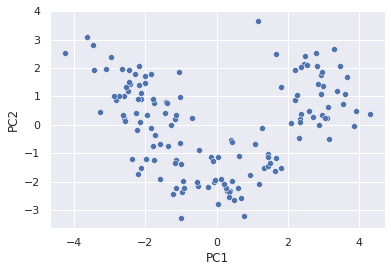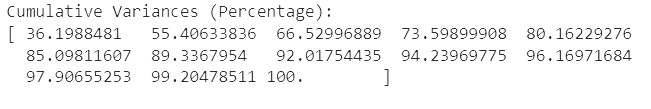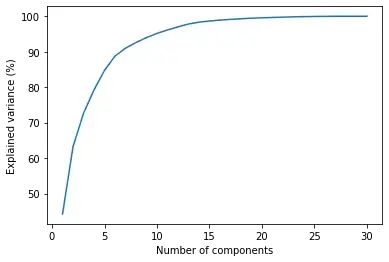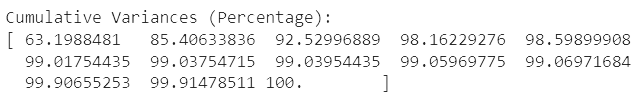Course Content

Principal Component AnalysisFit Data into the Model

Now that our data is ready, let's fit it into the PCA model.

We have reduced the dimension of the dataset from 13 characteristics to 2! Now we can visualize the resulting components using the seaborn library and matplotlib:It is logical, if you have a question, how to check the effectiveness of a particular PCA model. The performance of the PCA can be “counted” in two ways. The first is how much information the resulting components contain. The number of components that you decide to leave will determine how much information will eventually remain from the dataset. For example, let's display the amount of explained variance ratio:Above is the result of the PCA model, which contains 13 main components from the wine dataset (i.e. the same number of variables as it was originally). So, you can see that the first component captures 36% of the information, two components capture 55%, three components capture 66%, and so on.

The graph makes it easy to visualize the number of components required to capture varying degrees of data variability:The second way to evaluate the performance of a PCA model is to check the performance of other machine learning models into which you are going (if you really need to) fit the dataset. You can search for the optimal set of 3 variables - for example, the amount of time the machine learning model runs, the percentage of accuracy of the model, and the numbers of principal components.

## Quiz

Why do you think only 3 components in the presented dataset can explain as much as 92% of the data?